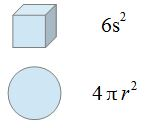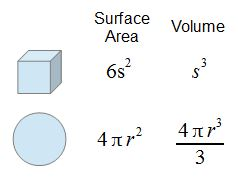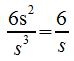# Surface Area to Volume Ratio

Lesson Transcript
Instructor: Yuanxin (Amy) Yang Alcocer

Amy has a master's degree in secondary education and has taught math at a public charter high school.

Although it may seem to be just a mathematical activity, the surface-area-to-volume ratio actually has real-world significance. In this lesson, you'll learn how you can calculate this ratio along with how useful this ratio is in various aspects of science.

## Surface Area

All shapes, including our bodies, have a surface area. A surface area is the area of the object that's exposed on the outside. So, for your body, it's how much skin you have. For a cube, it's the total area of all six sides of the cube. In math, geometric shapes have specific formulas you can use to calculate their surface areas.

A cube has a surface area of 6s2 and a sphere has a surface area of 4 * pi * r2.An error occurred trying to load this video.

Try refreshing the page, or contact customer support.

Coming up next: Properties of Shapes: Rectangles, Squares and Rhombuses

### You're on a roll. Keep up the good work!

Replay
Your next lesson will play in 10 seconds
• 0:04 Surface Area
• 0:32 Volume
• 1:15 Surface Area to Volume Ratio
• 1:44 Example
• 2:14 Lesson Summary
Save Save

Want to watch this again later?

Log in or sign up to add this lesson to a Custom Course.

Timeline
Autoplay
Autoplay
Speed Speed

## Volume

These same three-dimensional geometric shapes also have formulas for volume. The volume is the space inside the shape. Only three-dimensional shapes have volume. Two-dimensional shapes, such as a line or a square, don't have volume. If it helps, you can think of volume as how much liquid would fit inside a shape.

The formula for the volume of a cube is s3.

For the sphere, the volume is 4/3 * pi * r3.These formulas make it very easy to find the surface area and volume of these shapes. For other shapes, more complicated math such as integral calculus may be needed. For this lesson, though, you won't need to know calculus, just the formulas that are available for common shapes.

## Surface-Area-to-Volume Ratio

When you divide surface area by volume, you get a special ratio called the surface-area-to-volume ratio. This ratio can be noted as SA:V.To unlock this lesson you must be a Study.com Member.
Create your account

### Register to view this lesson

Are you a student or a teacher?

### Unlock Your Education

#### See for yourself why 30 million people use Study.com

##### Become a Study.com member and start learning now.
Back
What teachers are saying about Study.com
Create an account to start this course today
Used by over 30 million students worldwide